# BoxEdit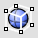Panels

BoxEdit

BoxEdit

The BoxEdit command edits objects according to their bounding box size, orientation, and position.

New bounding boxes are calculated each time the settings are applied.

#### Note

• When in individual transform mode, you can type a value (instead of scrolling) to set a value for all objects (absolute vs. relative).
• The edit controls can use units other than the model units.

#### Steps

1. Select objects.
2. Specify transform options.
3. Click Apply to accept the changes.
Or, click Reset to reject the changes.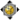Box Edit Panel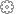Panel options

##### n objects selected

Reports the number of objects selected.

##### Apply

Save the changes.

##### Reset

Rejects the changes and revert the objects to their previous state.

#### Size

Use any units to specify sizes.

##### X/Y/Z

Specifies the x, y, or z size in model units.

##### Uniform

Sizes all three coordinates using the same value.

##### Increment

Increment used by the spinner controls.

#### Scale

##### X/Y/Z

Specifies the x, y, or z scale factor.

##### Uniform

Sizes all three coordinates using the same value.

##### Increment

Increment used by the spinner controls.

#### Position

##### X/Y/Z

Specifies the x, y, or z position in model units.

##### Uniform

Sizes all three coordinates using the same value.

##### Increment

Increment used by the spinner controls.

#### Rotation

##### X/Y/Z

Specifies the x, y, or z rotation in degrees.

##### Uniform

Sizes all three coordinates using the same value.

##### Increment

Increment used by the spinner controls.

Note: The precision of the x, y, z values in Size, Scale, Position and Rotation are controlled by Unit Display Precision.

#### Options

##### X/Y/Z

Specifies which axis to use to set the direction being used for uniform transforms.

##### Pivot location

Sets the base point for scale and rotate transforms.

##### Use bounding boxes

Uses the bounding box of all the selected objects or the bounding box of individual objects when Transform individual objects is checked.

##### Use gumball

Uses the gumball pivot point to transform objects.

##### Block instances use insertion points

Forces objects to be transformed individually. Selected objects that are not blocks use individual bounding boxes.

##### X/Y/Z

Sets the x, y, or z location.

##### Min

Uses the minimum bounding box x, y, or z point.

Set X, Y, and Z to Min to use the "lower-left" corner of the bounding box as the pivot.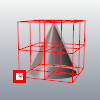Minimum x, y, and z.
##### Cen

Uses the centroid bounding box x, y, or z point.

Set X, Y, and Z to Cen to use the "middle" of the bounding box as the pivot.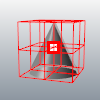Center x, y, and z.
##### Max

Uses the maximum bounding box x, y, or z point.

Set X, Y, and Z to Max to use the "upper-right" corner of the bounding box as the pivot.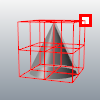Maximum x, y, and z.
##### Use world CPlane

Uses world coordinates for the transformations.

##### Use current CPlane

Uses construction plane coordinates for the transformations.

##### Transform objects individually

Transforms each object from its own pivot point and bounding box.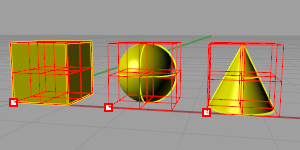Individual bounding boxes and pivot for each object.

By default, selected objects are all contained in one bounding box and are transformed from one pivot point as one unit.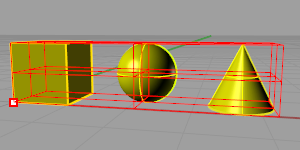Single bounding box and pivot for all selected objects.
##### Show bounding box

Displays a control polygon that represents the bounding box.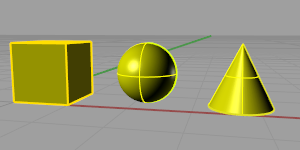Bounding box off.
##### Copy objects

Transforms a copy instead of the original objects.

##### Select copied objects

Leaves the copied objects selected. This allows incremental changes to the copied objects.